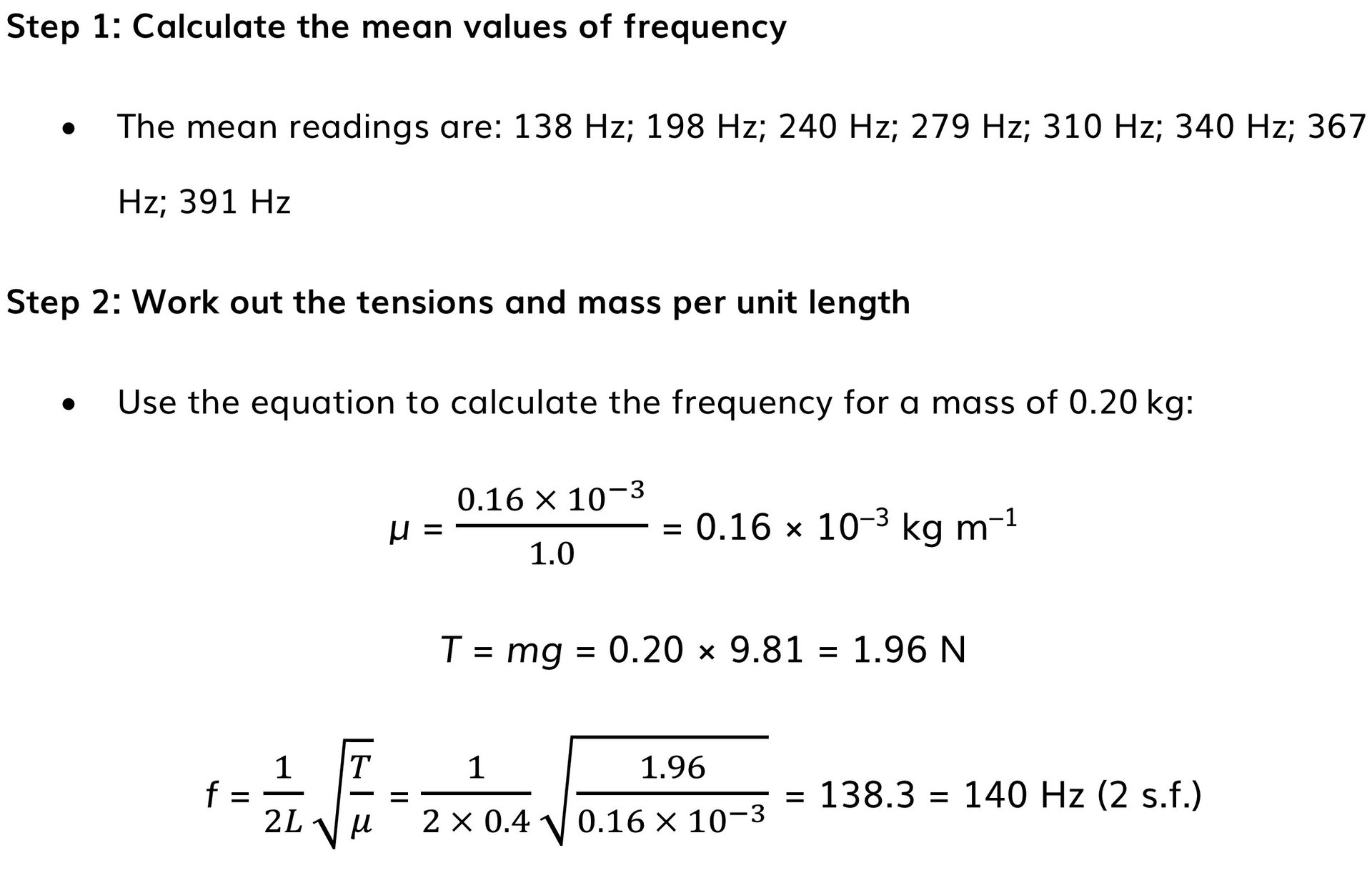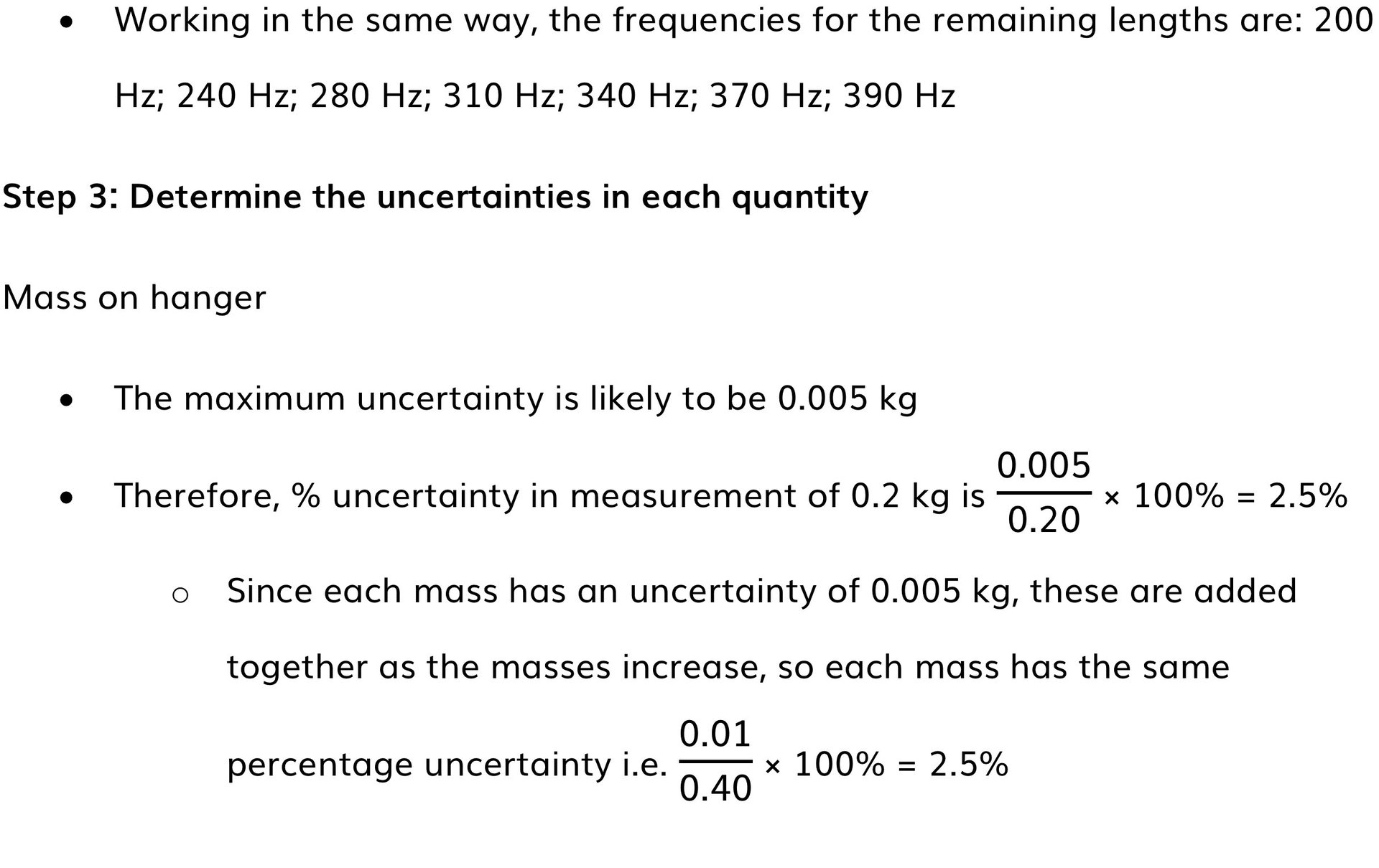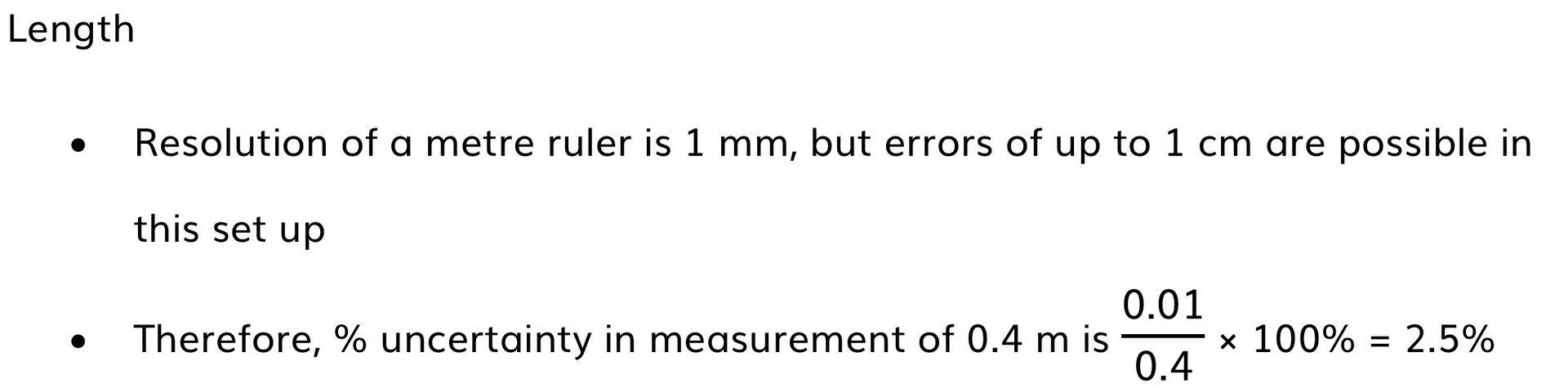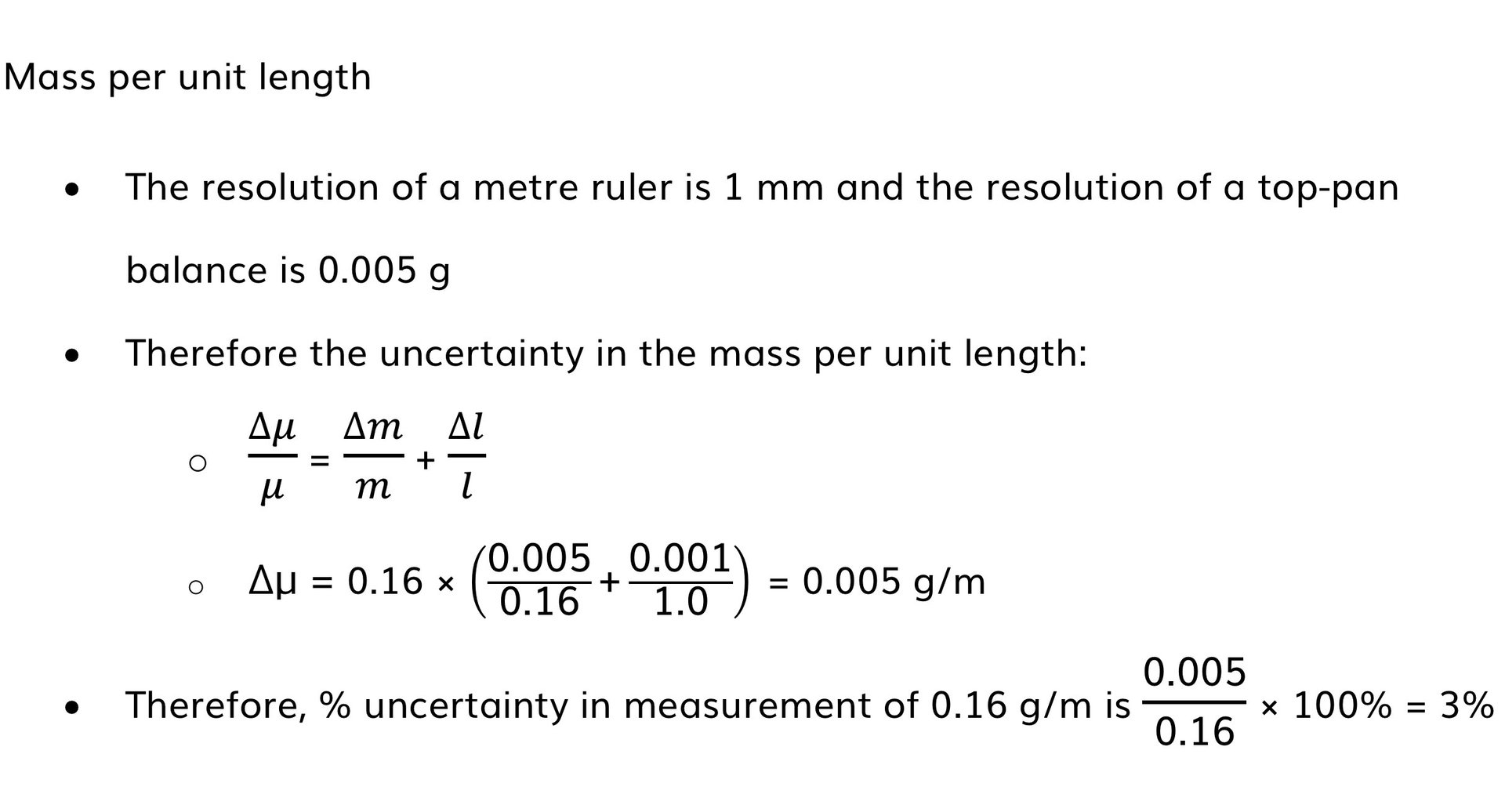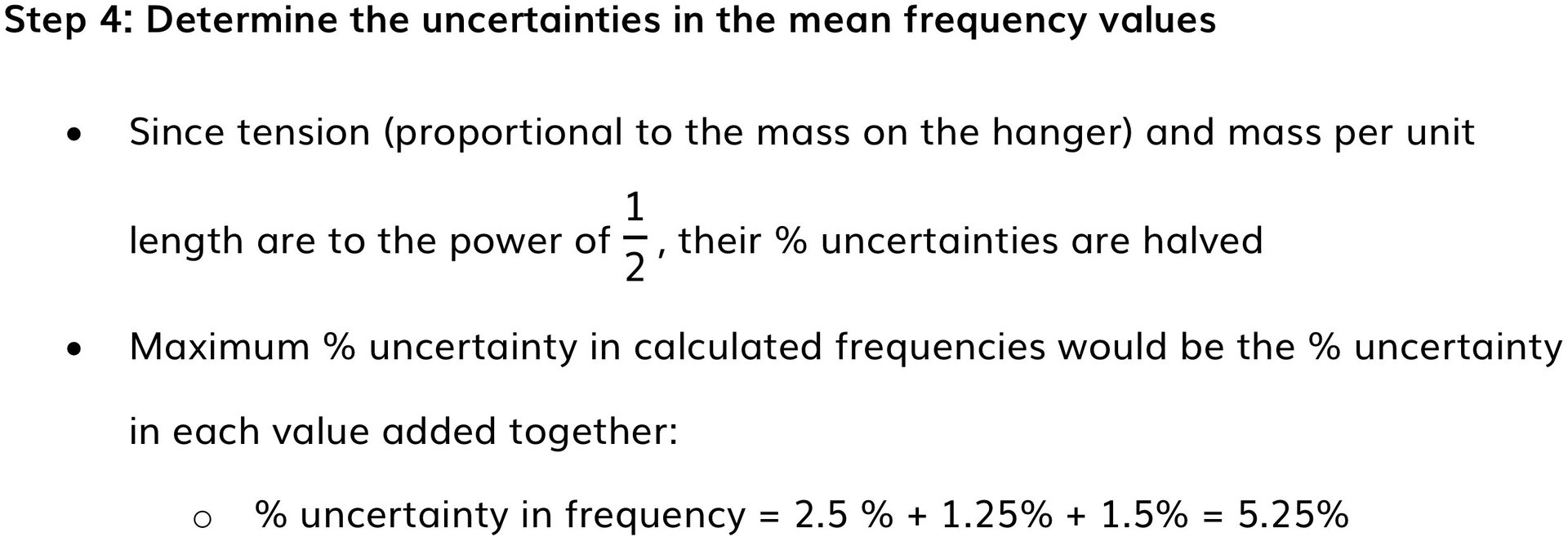# AQA A Level Physics复习笔记3.2.4 Required Practical: Investigating Stationary Waves

### Required Practical: Investigating Stationary Waves

#### Aims of the Experiment

• The overall aim of the experiment is to measure how the frequency of the first harmonic is affected by changing one of the following variables:
• The length of the string
• The tension in the string
• Strings with different values of mass per unit length

Variables:

• Independent variable = either length, tension, or mass per unit length
• Dependent variable = frequency of the first harmonic
• Control variables
• If length is varied = same masses attached (tension), same string (mass per unit length)
• If tension is varied = same length of the string, same string (mass per unit length)
• If mass per unit length is varied = same masses attached (tension), same length of the string

#### Equipment List• Resolution of measuring equipment:
• Metre ruler = 1 mm
• Signal generator ~ 10 nHz
• Top-pan balance = 0.005 g

#### Method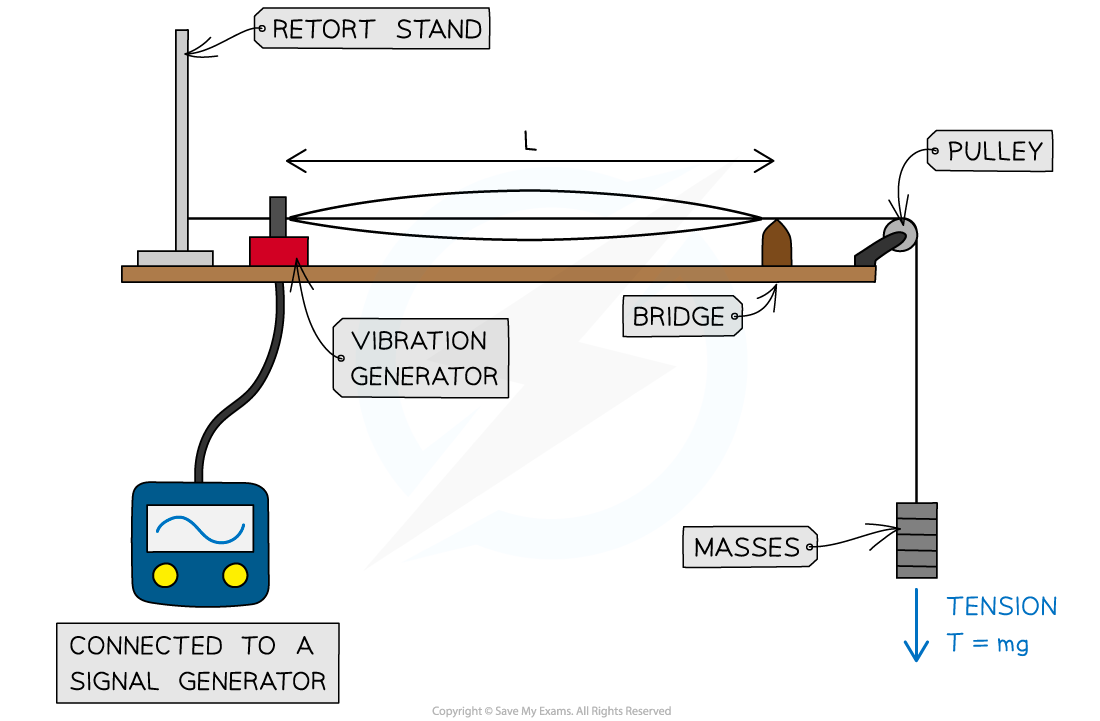The setup of apparatus required to measure the frequency of the first harmonic at different values of length, tension, or mass per unit length

This method is an example of the procedure for varying the length of the string with the frequency – this is just one possible relationship that can be tested

1. Set up the apparatus by attaching one end of the string to the vibration generator and pass the other end over the bench pulley and attach the mass hanger
2. Adjust the position of the bridge so that the length L is measured from the vibration generator to the bridge using a metre ruler
3. Turn on the signal generator to set the string oscillating
4. Increase the frequency of the vibration generator until the first harmonic is observed and read the frequency that this occurs at
5. Repeat the procedure with different lengths
6. Repeat the frequency readings at least two more times and take the average of these measurements
7. Measure the tension in the string using T = mg
• Where m is the amount of mass attached to the string and g is the gravitational field strength on Earth (9.81 N kg–1)

8. Measure the mass per unit length of the string μ = mass of string ÷ length of string
• Simply take a known length of the string (1 m is ideal) and measure its mass on a balance

• An example of a table with some possible string lengths might look like this: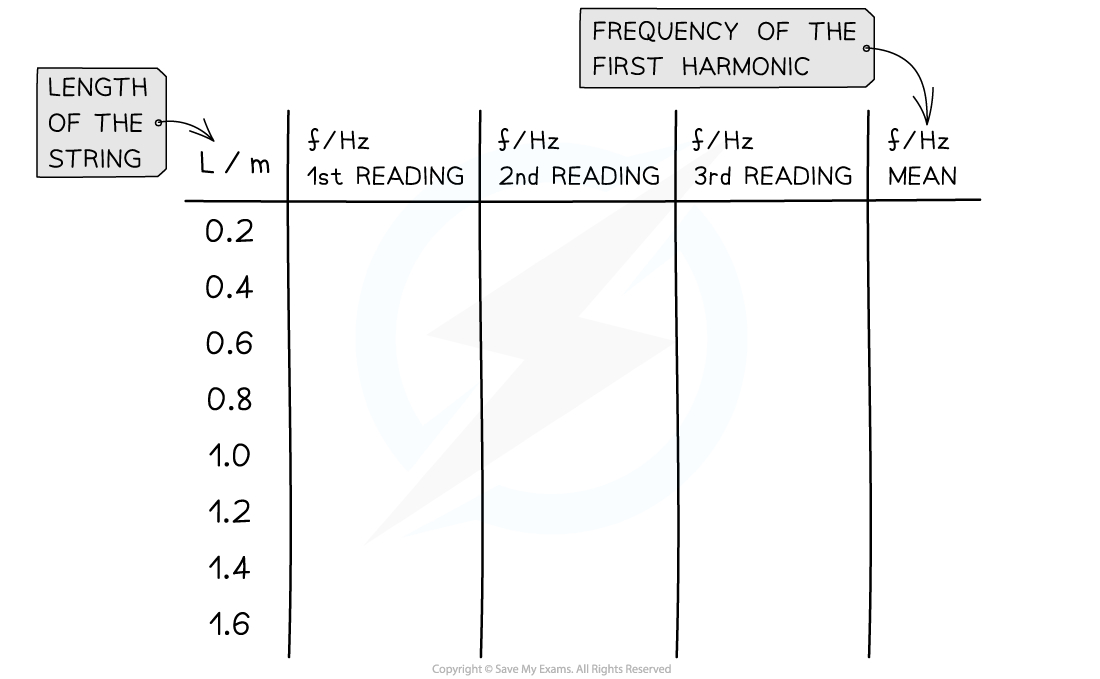Before conducting an experiment, a table must be set up to detail of what measurements are to be made

#### Analysing the Results

• For the first harmonic, wavelength, λ = 2L
• So, the speed of the stationary wave is:

v = fλ = f × 2L

• Rearranging for frequency, f:• Comparing this to the equation of a straight line: y = mx
• y = f (Hz)
• x = 1/L (m–1)
• Gradient = v/2 (m s–1)

1. Plot a graph of the mean values of f against 1/L
2. Draw a line of best fit and calculate the gradient
3. Work out the wave speed, which will be 2 × gradient:If the frequency is plotted again the inverse of the length, the velocity is twice the gradient of the graph

• Verify the wave speed of the travelling waves using the equation:• Where:
• T = tension (N)
• μ = mass per unit length (kg m−1)

• Assess the uncertainties in the measurements of length and the frequency and carry out calculations to determine the uncertainty in the wave speed

#### Evaluating the Experiment

Systematic errors:

• An oscilloscope can be used to verify the signal generator’s readings
• The signal generator should be left for about 20 minutes to stabilise
• The measurements would have a greater resolution if the length used is as large as possible, or as many half-wavelengths as possible
• This means measurements should span a suitable range, for example, 20 cm intervals over at least 1.0 m

Random errors:

• The sharpness of resonance leads to the biggest problem in deciding when the first harmonic is achieved
• This can be resolved by adjusting the frequency while looking closely at a node. This is a technique to gain the largest response
• Looking at the amplitude is likely to be less reliable since the wave will be moving very fast

• When taking repeat measurements of the frequency, the best procedure is as follows:
• Determine the frequency of the first harmonic when the largest vibration is observed and note down the frequency at this point
• Increase the frequency and then gradually reduce it until the first harmonic is observed again and note down the frequency of this
• If taking three repeat readings, repeat this procedure again
• Average the three readings and move onto the next measurement

#### Safety Considerations

• Use a rubber string instead of a metal wire, in case it snaps under tension
• If using a metal wire, wear goggles to protect the eyes in case it snaps
• Stand well away from the masses in case they fall onto the floor
• Place a crash mat or any soft surface under the masses to break their fall

#### Worked Example

A student investigates the relationship between the frequency of the stationary waves on a wire and the tension in the wire. The tension is varied by adding masses to a hanger which is attached to a pulley over one end of a table.The student records the following data:

• Mass of the wire = 0.16 g
• Length of the wire weighed = 1.0 m
• Distance between the fixed ends = 0.4 m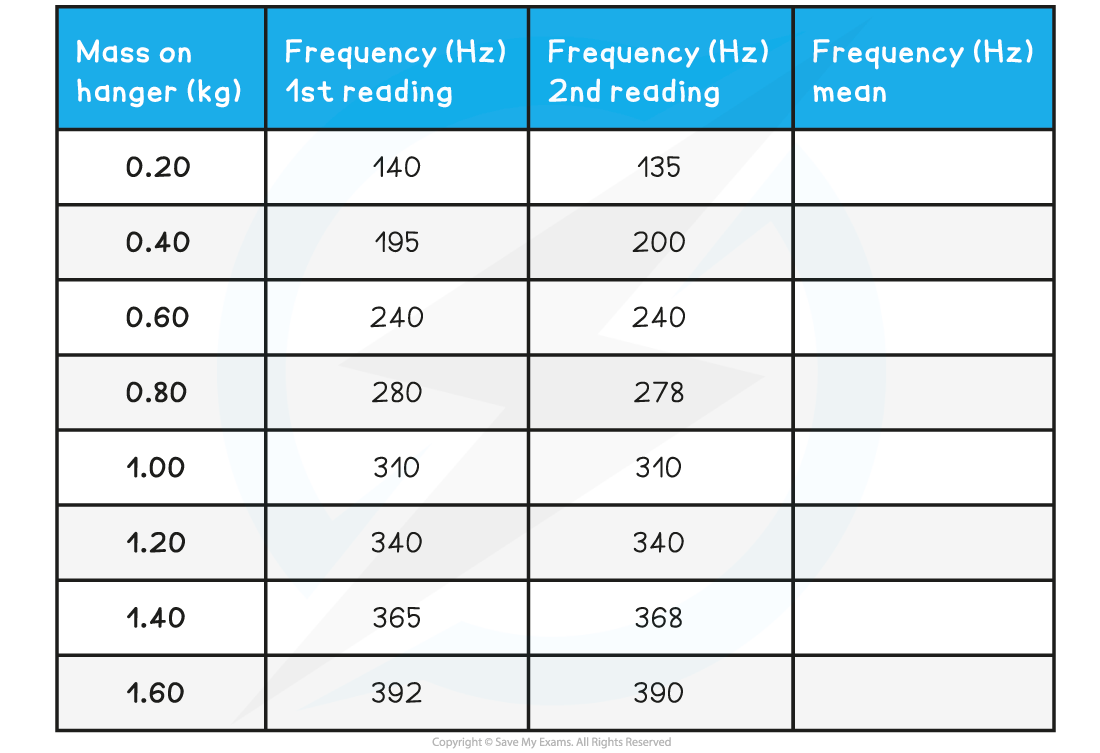Calculate the frequency using the relation between frequency, length, tension and mass per unit length. Evaluate the percentage uncertainty in these values.